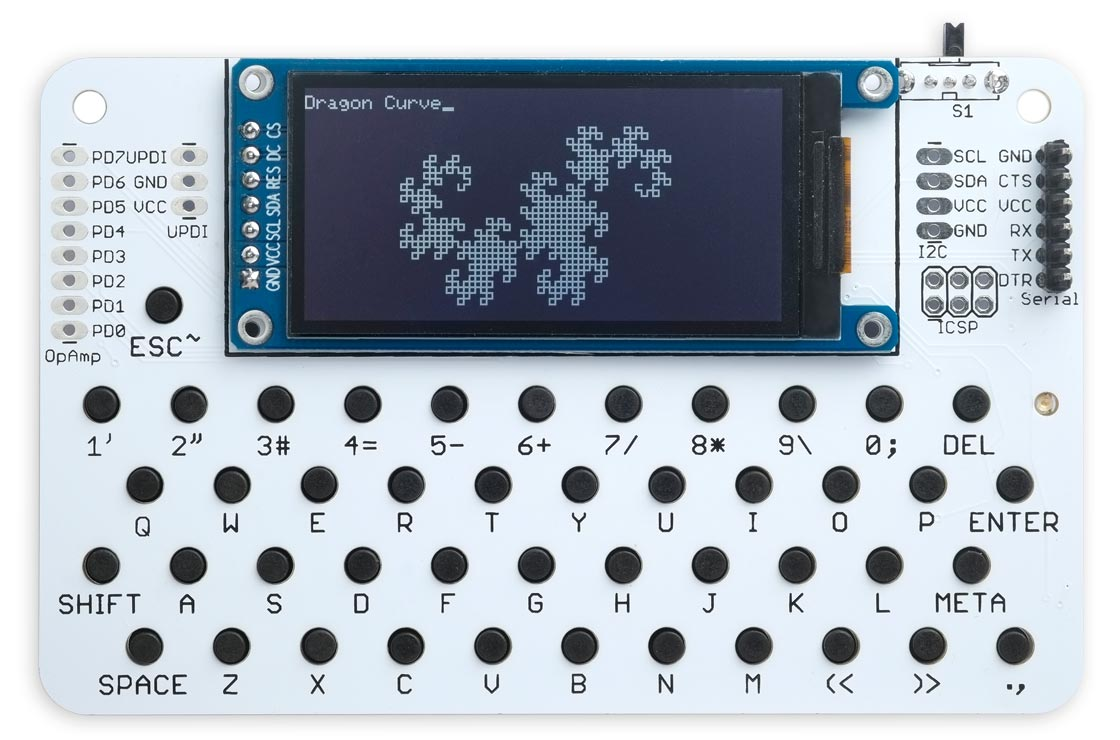## Dragon curve

27th September 2023

This recursive function plots a fractal curve known as the Dragon Curve :It's shown above running on the Lisp Badge LE.

Here's the program:

```;
; Dragon Curve

(defvar *x*)
(defvar *y*)
(defvar *th*)

(defun forward (length)
(let ((new-x (+ *x* (* length (cos *th*))))
(new-y (- *y* (* length (sin *th*)))))
(draw-line *x* *y* new-x new-y)
(setf *x* new-x)
(setf *y* new-y)))

(defun sin (th) (nth th '(0 1 0 -1)))
(defun cos (th) (nth th '(1 0 -1 0)))

(defun rotate (angle) (setf *th* (mod (+ *th* angle) 4)))

(defun dragon (size level turn)
(cond
((zerop level) (forward size))
(t
(dragon size (1- level) 1)
(rotate turn)
(dragon size (1- level) -1))))

(defun go ()
(setq *x* 80)
(setq *y* 50)
(setq *th* 3)
(write-byte 12)
(princ "Dragon Curve")
(dragon 3 10 1)
(loop))```

It uses Turtle Graphics, but since it only uses right angle turns it doesn't need floating point for the calculations, and so will run on any version of uLisp.

1. ^ Dragon curve on Wikipedia.